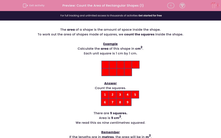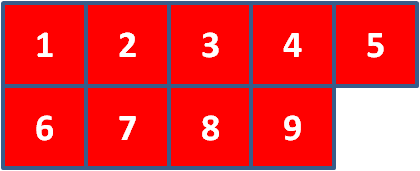# Count the Area of Rectangular Shapes (1)

In this worksheet, students must calculate the area of rectilinear shapes made of cm or m squares by counting the squares.Key stage:  KS 2

Curriculum topic:   Maths and Numerical Reasoning

Curriculum subtopic:   Perimeter and Area

Popular topics:   Area worksheets

Difficulty level:#### Worksheet Overview

The area of a shape is the amount of space inside the shape.

To work out the area of shapes made of squares, we count the squares inside the shape.

Example

Calculate the area of this shape in cm2.

Each unit square is 1 cm by 1 cm.Count the squares.There are 9 squares.

Area is 9 cm2.

We read this as nine centimetres squared.

Remember

If the lengths are in metres, the area will be in m2.

### What is EdPlace?

We're your National Curriculum aligned online education content provider helping each child succeed in English, maths and science from year 1 to GCSE. With an EdPlace account you’ll be able to track and measure progress, helping each child achieve their best. We build confidence and attainment by personalising each child’s learning at a level that suits them.

Get started